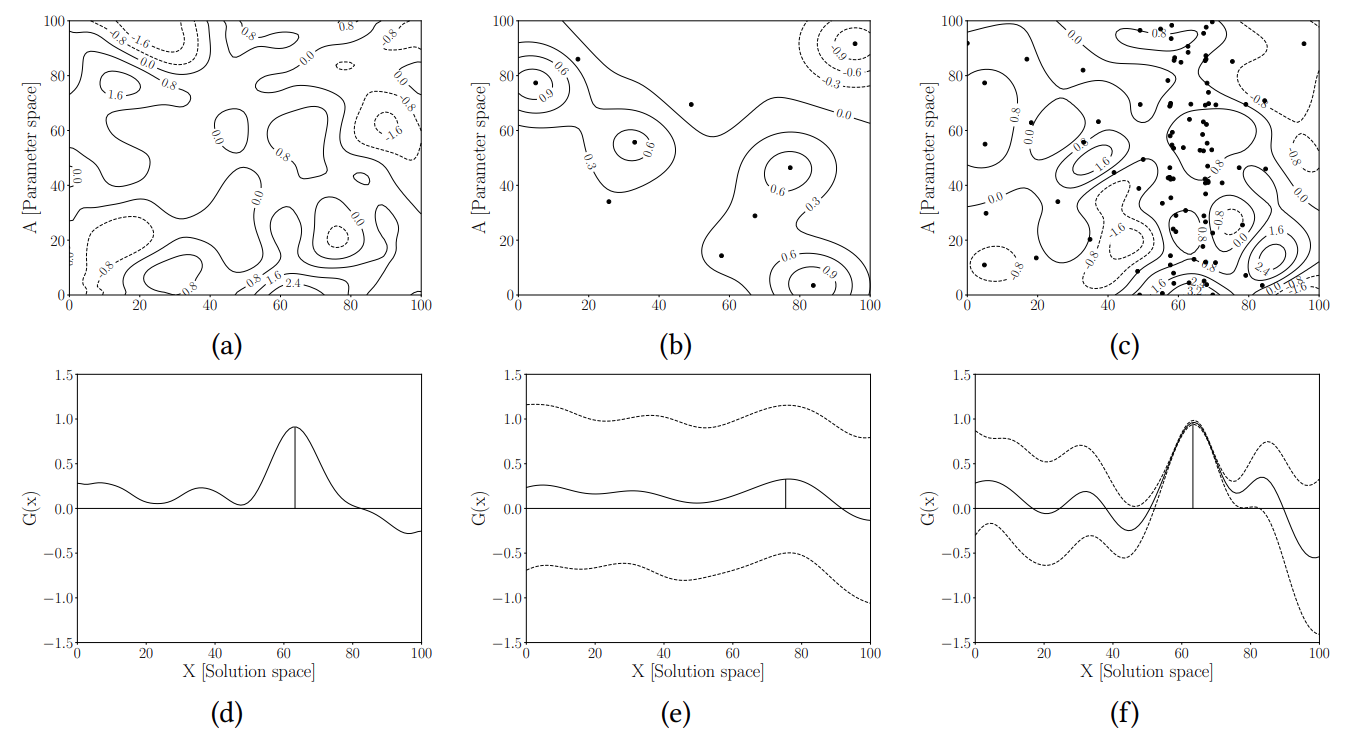Bayesian Optimisation vs. Input Uncertainty Reduction

2021, Jan 03

This is the work of Juan Ungredda Google Scholar, Student Homepage

Problem Setting

In simulation optimization, we try to optimize real world problems, queing systems, productions lines, traffics lights, by using computer simulations (instead of playing with real systems in the real world directly) as they are faster and less risky. Optimization algorithms often applied ot these simualtions to make find the best possible setting that can then be used in the real world. However, these simulators require calibration, tuning to a given applicaiton.

Mathematically, we assume the simulator is a simply an expensive black box function with two arguments f(x, a), a decision variable to optimize, x, as well as a calbration parameter, a. We want to collect real world data to tune the callibration parameter a and make the simulator f(x, a) an accurate duplicate of the real world as possible, find a*. We also want to search for the best x* that is the best input to the simulator

x* = argmax f(x, a*)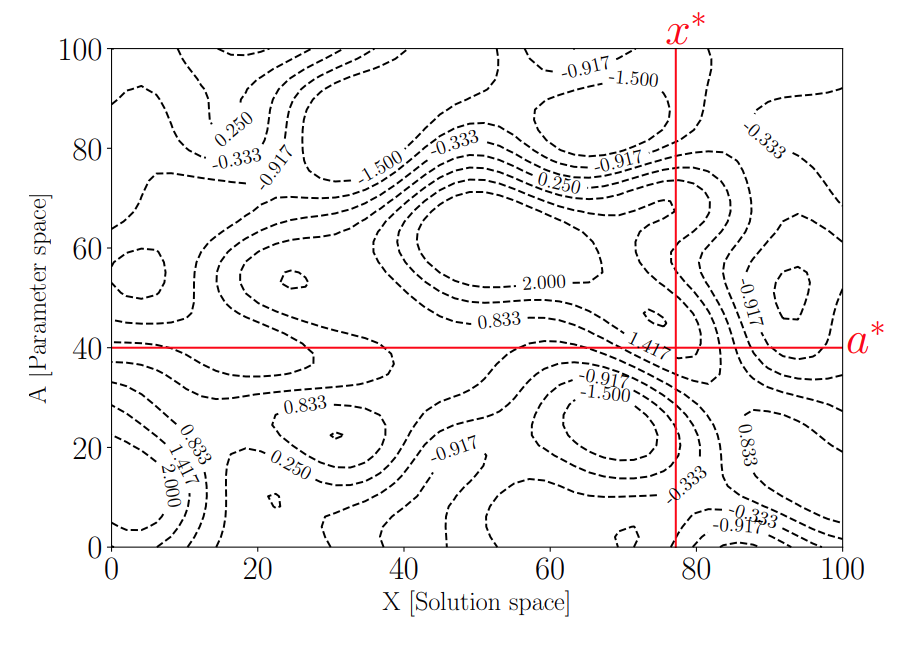However there is uncertainty about the true a*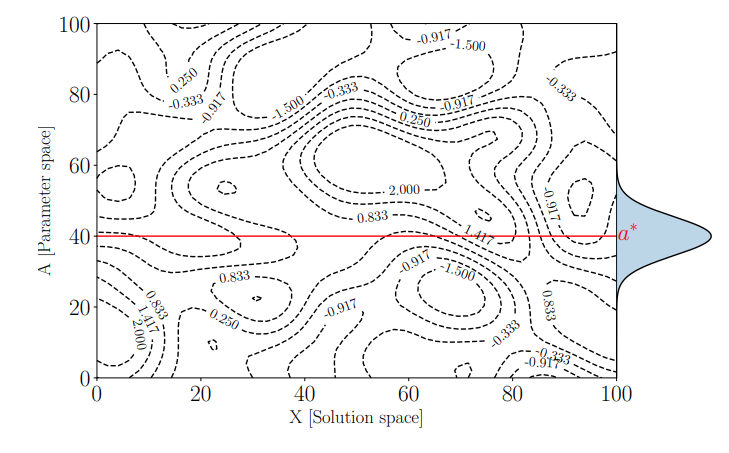and there is uncertianty about the actual function f(x, a) as modelled by a Gaussian Process.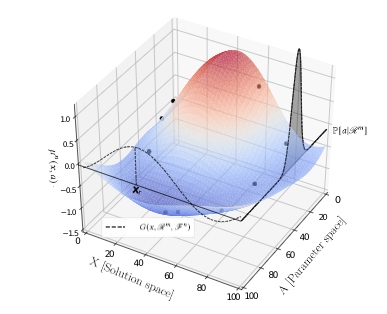We can collect data to learn more about a* by looking at how the distributuion for a would change if we collect more data, one step look ahead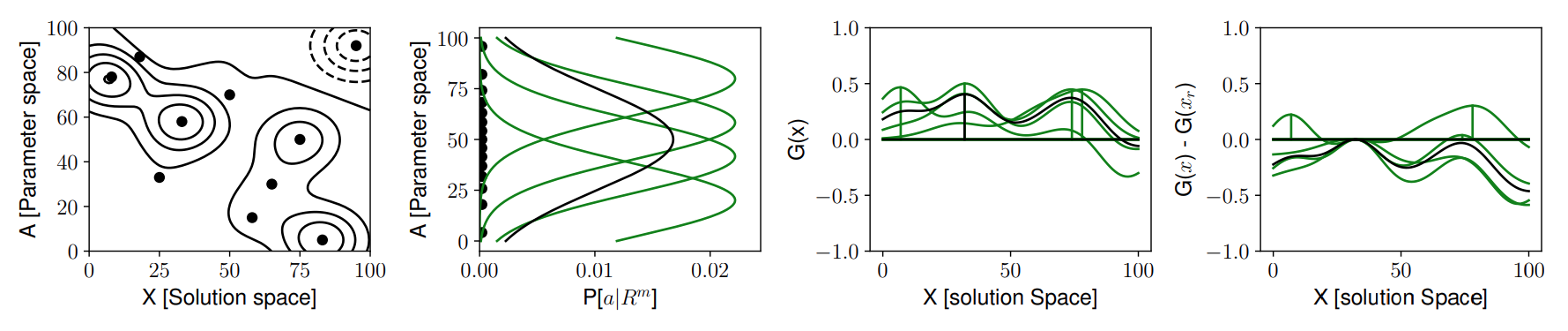or we may evaluate the simulator f(x, a) at a given point (x, a) and update the model of f(x, a)